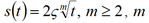##### On Finding Geodesic Equation of Two Parameters Inverse Gaussian Distribution

William W. S. Chen

Journal of Advances in Mathematics and Computer Science, Page 1-8
DOI: 10.9734/BJMCS/2016/24577

The class of Inverse Gaussian distributions is quite commonly used as a life-time model in reliability studies. The books by Chhikara and Folks  and Seshadri  present extensive discussions on classical inference for the parameters of Inverse Gaussian distribution.

However, in this paper, we switch our attention to find its geodesic equation. We applied two different algorithms to solve some partial differential equations, where these equations originated from the Inverse Gaussian distribution. As expected, the two algorithms yield the same result.

##### Oscillatory Blood Flow in Convergent and Divergent Channels, Part 2: Effects of Reynolds Number

W. I. A. Okuyade, T. M. Abbey

Journal of Advances in Mathematics and Computer Science, Page 1-14
DOI: 10.9734/BJMCS/2016/23222

This paper studies the effects of Reynolds number on the oscillating flow in convergent and divergent channels. The nonlinear equations governing the flow are solved analytically by the method of perturbation series solutions. Expressions for the velocities and wall shear stress are obtained and analyzed graphically. It is found that increase in the Reynolds number increases the velocities and wall shear stress. Similarly, it is seen that a flow separation occurs in the radial velocity flow structure.

##### An Information-Theoretic Alternative to the Cronbach's Alpha Coecient of Item Reliability

E. Fokoué, N. Gündüz

Journal of Advances in Mathematics and Computer Science, Page 1-9
DOI: 10.9734/BJMCS/2016/23944

We propose an information-theoretic alternative to the popular Cronbach alpha coefficient of item reliability. Particularly suitable for contexts in which instruments are scored on a strictly nonnumeric and non ordinal scale, our proposed index is based on functions of the entropy of the empirical distributions defined on the sample space of responses. Our proposed reliability index tracks the Cronbach alpha coefficient uniformly while offering several other advantages discussed in great details in this paper.

##### Mathematical Study of In-host Dynamics of Hepatitis B Virus in Absence of Immunity System

Jannatun Nayeem, Chandra N. Podder

Journal of Advances in Mathematics and Computer Science, Page 1-21
DOI: 10.9734/BJMCS/2016/23120

We develop a mathematical model to understand the dynamics of HBV-in host infection of individuals in vivo. The model incorporates the uninfected host cells, short lived infected cells, chronically infected cells, free virus particles, humoral immune response of HBV specific antibodies and cell mediated immune response of CTLs is analysed to gain its characteristic within human cell mechanism. At first we have analyzed the stability analysis of host cells and infected cells without the effect of immunity system and also discuss the graphical analysis with immunity system. Present study represents a mathematical model, which exhibit two equilibrium points namely, the virus free equilibrium (VFE) and virus present equilibrium (VPE). It is found that using Lyapunov function the virus free equilibrium (VFE) is globally asymptotically stable (GAS) when R0 < 1. And also the virus present equilibrium point (VPE) is locally asymptotically stable when R0 > 1.

##### Comparison of Least Squares Method and Bayesian with Multivariate Normal Prior in Estimating Multiple Regression Parameters

Felix O. Mettle, Louis Asiedu, Enoch N. B. Quaye, Abeku A. Asare-Kumi

Journal of Advances in Mathematics and Computer Science, Page 1-8
DOI: 10.9734/BJMCS/2016/23145

Based on an assumption of multivariate normal priors for parameters of multivariate regression model, this study outlines an algorithm for application of traditional Bayesian method to estimate regression parameters. From a given set of data, a Jackknife sample of least squares regression coefficient estimates are obtained and used to derive estimates of the mean vector and covariance matrix of the assumed multivariate normal prior distribution of the regression parameters. Driven to determine whether Bayesian methods to multivariate regression parameter estimation present a stable and consistent improvement over classical regression modeling or not, the study results indicate that the Bayesian method and Least Squares Method (LSM) produced almost the same estimates for the regression parameters and coefficient of determination (to 4.dp) with the Bayesian method having smaller standard errors.

##### A Numerical Method Based Boundary Integral Equation to Solve Multiphase Moving Boundary Problems

S. G. Ahmed, M. Saif AlDien, M. Y. Youssef

Journal of Advances in Mathematics and Computer Science, Page 1-10
DOI: 10.9734/BJMCS/2016/23880

The present paper deals with moving boundary problems by fixing the moving boundary by an assumption of the formis assumed power and ς is an unknown. An iterative algorithm is then developed within the main algorithm to solve the phases that appear throughout the m whole process with moving boundaries at each time step as phases with fixed boundaries. A two test problems are solved using the present method. The results due to the first test problem were compared with previous numerical results based on boundary integral formulation, while the results due to the second one was compared with available analytical solution. An overall good agreement is obtained for both two examples compared with the previous numerical and analytical results.

##### Graph Compositions of Uniform Four q-fans

E. Mphako-Banda, R. Kwashira, B. Zinsou

Journal of Advances in Mathematics and Computer Science, Page 1-15
DOI: 10.9734/BJMCS/2016/24264

Graph compositions play an important role in the generalization of both ordinary compositions of positive integers and partitions of nite sets. Graph compositions of certain classes of graphs, like trees, cycle graphs, wheels, etc have been found using generating functions and recurrence relations. In this paper, we use di erent combinatorial techniques, to count the number of graph compositions of uniform four q-fans.